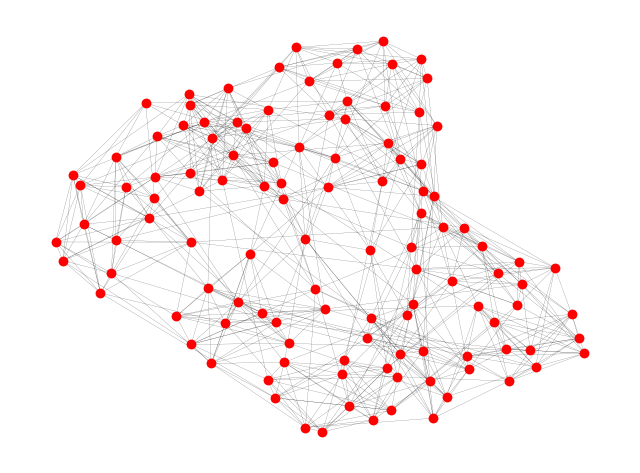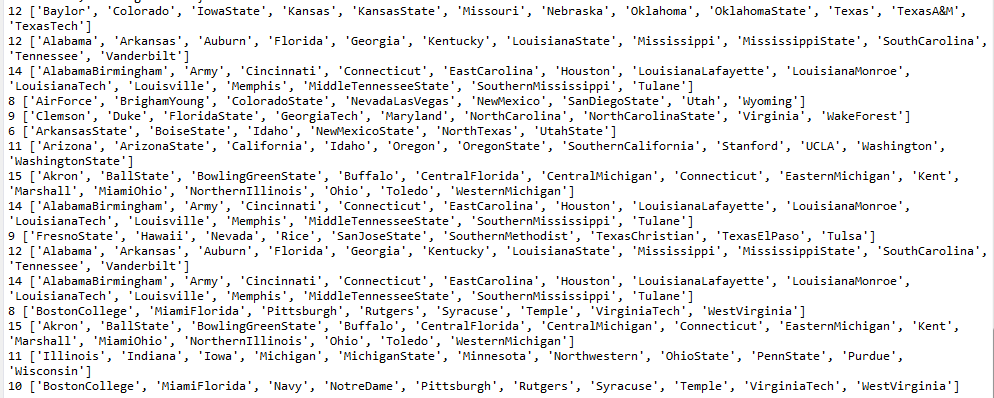• 社区发现算法python
2021-09-20 14:18:02

算法是以供水管网数据为例，后面给出了社区发现结果评价指标，模块度的计算结果。如有代码疑问，私聊即可。
注：代码本人手写，未考虑任何效率问题，只是将算法过程实现，关于算法的介绍需要自行查看。
SCAN算法中重要的两个参数为密度阈值（对标DBSCAN中的min_pts），节点结构相似度阈值（对标DBSCAN的邻域半径）。其中节点结构相似度阈值范围【0，1】，密度阈值最好大于等于2。

import wntr as wr
from plotly import *
import networkx as nx
import matplotlib.pyplot as plt
import pandas as pd
import numpy as np

wdn = wr.network.WaterNetworkModel("管网.inp")
# 转化为图
wdnG = wdn.get_graph()
# 无向图
uG = wdnG.to_undirected()
# 单边图
# sG = nx.Graph(uG)

def Similarity(obj1, obj2):
list1 = set([])
list2 = set([])
for i in uG[obj1].keys():
for m in uG[obj2].keys():
return len(list1 & list2)/len(list1 | list2)

# 邻域半径e
def retrieve_neighbors(center_index, e):
neighborhood = []
for i in wdn.node_name_list:
if Similarity(center_index, i) >= e:
neighborhood.append(i)
neighborhood.remove(center_index)
return neighborhood

# 密度阈值u
def SCAN(u, e):
cluster_label = 0
non_member = -1
unmarked = 9999
stack = []
min_neighbors = u
for i in wdn.node_name_list:
wdn.nodes[i].cluster = unmarked
for j in wdn.node_name_list:
if wdn.nodes[j].cluster == unmarked:
neighborhood = retrieve_neighbors(j, e)
if len(neighborhood) < min_neighbors:
wdn.nodes[j].cluster = non_member
else:
cluster_label += 1
wdn.nodes[j].cluster = cluster_label
for k in neighborhood:
wdn.nodes[k].cluster = cluster_label
stack.append(k)
while len(stack) > 0:
current_core_index = stack.pop()
new_neighborhood = retrieve_neighbors(current_core_index, e)
if len(new_neighborhood) >= min_neighbors:
for m in new_neighborhood:
if any([wdn.nodes[m].cluster == non_member,
wdn.nodes[m].cluster ==unmarked]):
wdn.nodes[m].cluster = cluster_label
stack.append(m)
if cluster_label > 1:
community = [[] for single in range(0, cluster_label)]
for temp in wdn.node_name_list:
community[wdn.nodes[temp].cluster - 1].append(temp)
return wdn, cluster_label, community
else:
return wdn, cluster_label, [wdn.node_name_list]

print(nx.algorithms.community.modularity(SCAN(4, 0.2).get_graph(), SCAN(4, 0.2)))python
更多相关内容
• 基于LFM的重叠社区发现算法python代码实现 import random import networkx as nx import matplotlib.pyplot as plt import zipfile #import urllib.request as urllib class Community(): ''' use set operation to...

## 基于LFM的重叠社区发现算法python代码实现

import random
import networkx as nx
import matplotlib.pyplot as plt
import zipfile
#import urllib.request as urllib
class Community():
''' use set operation to optimize calculation '''

def __init__(self,G,alpha=1.0):
self._G = G
self._alpha = alpha
self._nodes = set()
self._k_in = 0
self._k_out = 0
neighbors = set(self._G.neighbors(node))
#print("添加令居节点",neighbors , self._nodes,neighbors & self._nodes)
node_k_in = len(neighbors & self._nodes)#neighbor和self._nodes公有节点的数目存入node_k_in
#print("node_k_in",node_k_in)
node_k_out = len(neighbors) - node_k_in
#print("node_k_out",node_k_out)
self._k_in += 2*node_k_in
self._k_out = self._k_out+node_k_out-node_k_in

def remove_node(self,node):
neighbors = set(self._G.neighbors(node))
community_nodes = self._nodes
#print("community_nodes",community_nodes)
node_k_in = len(neighbors & community_nodes)
node_k_out = len(neighbors) - node_k_in
self._nodes.remove(node)
self._k_in -= 2*node_k_in
self._k_out = self._k_out - node_k_out+node_k_in

neighbors = set(self._G.neighbors(node))
old_k_in = self._k_in
old_k_out = self._k_out
vertex_k_in = len(neighbors & self._nodes)#vertex顶点
vertex_k_out = len(neighbors) - vertex_k_in
new_k_in = old_k_in + 2*vertex_k_in
new_k_out = old_k_out + vertex_k_out-vertex_k_in
new_fitness = new_k_in/(new_k_in+new_k_out)**self._alpha#幂次
old_fitness = old_k_in/(old_k_in+old_k_out)**self._alpha
return new_fitness-old_fitness

def cal_remove_fitness(self,node):
neighbors = set(self._G.neighbors(node))
new_k_in = self._k_in
new_k_out = self._k_out
node_k_in = len(neighbors & self._nodes)
node_k_out = len(neighbors) - node_k_in
old_k_in = new_k_in - 2*node_k_in
old_k_out = new_k_out - node_k_out + node_k_in
old_fitness = old_k_in/(old_k_in+old_k_out)**self._alpha
new_fitness = new_k_in/(new_k_in+new_k_out)**self._alpha
return new_fitness-old_fitness

def recalculate(self):
for vid in self._nodes:
fitness = self.cal_remove_fitness(vid)
if fitness < 0.0:
return vid
return None

def get_neighbors(self):
neighbors = set()
for node in self._nodes:
neighbors.update(set(self._G.neighbors(node)) - self._nodes)
return neighbors

def get_fitness(self):
return float(self._k_in)/((self._k_in+self._k_out) ** self._alpha)

class LFM():

def __init__(self, G, alpha):
self._G = G
self._alpha = alpha

def execute(self):
communities = []
print("嘿嘿",list(self._G.node.keys()))
print("---------------------")
node_not_include = list(self._G.node.keys())
while(len(node_not_include) != 0):
c = Community(self._G, self._alpha)
#print("self._alpha",self._alpha)#0.9
# randomly select a seed node
seed = random.choice(node_not_include)
print("随机选取节点是：",seed)
to_be_examined = c.get_neighbors()
print("c.get_neighbors()",c.get_neighbors())
while(to_be_examined):
#largest fitness to be added
m = {}
for node in to_be_examined:
m[node] = fitness
to_be_add = sorted(m.items(),key=lambda x:x,reverse = True)#啥意思？？？
#适应度降序排列
#stop condition
break
to_be_remove = c.recalculate()
while(to_be_remove != None):
c.remove_node(to_be_remove)
to_be_remove = c.recalculate()

to_be_examined = c.get_neighbors()

for node in c._nodes:
if(node in node_not_include):
node_not_include.remove(node)
communities.append(c._nodes)
return communities

if(__name__ == "__main__"):
#G = nx.karate_club_graph()#一个边集一个点集
# G = nx.florentine_families_graph()
zf = zipfile.ZipFile('football.zip')  # zipfile object
txt = zf.read('football.txt').decode()  # read info file
gml = zf.read('football.gml').decode()  # read gml data
# throw away bogus first line with # from mejn files
gml = gml.split('\n')[1:]
G = nx.parse_gml(gml)  # parse gml data

print(txt)
# print degree for each team - number of games
for n, d in G.degree():
print('%s %d' % (n, d))

options = {
'node_color': 'red',
'node_size': 50,
'line_color': 'grey',
'linewidths': 0,
'width': 0.1,
}
nx.draw(G, **options)
#networkx.draw(G, with_labels=True)
plt.show()
algorithm = LFM(G,0.9)
communities = algorithm.execute()
for c in communities:
print (len(c),sorted(c))


社区网络：重叠社区划分结果：展开全文机器学习
• LFM算法是来源于论文《Detecting the overlapping and hieerarchical community structure in complex networks》，文档中包含该算法python的源码，以及用到的数据集，仅供大家学习参考。
• 社区发现FN算法Python实现，并与GN算法在结果优劣以及计算效率方面进行对比。

### 社区发现FN算法Python实现

​2004年，Newman在GN(Girvan and Newman, 2002)算法的基础上，提出了另外一种快速检测社区的算法，称为FN算法。该算法能得到和GN算法相似的结构，但是时间复杂度更低，GN算法的时间复杂度为 O ( m 2 n ) O(m^2n) ，FN算法的时间复杂度为 O ( ( m + n ) n ) O((m+n)n) ，其中， m m 是边的数量， n n 是节点的数量。此处给出FN算法的Python实现，并给出对比实验以及社区发现的三种评价指标。Newman, M. E. J. ,2004. Fast algorithm for detecting community structure in networks. phys rev e stat nonlin soft matter phys, 69, 066133.

去看原文

## 算法原理

FN算法是一种层次聚类算法。起初每个节点都是一个类。每次合并让Q值增加（即 Δ Q \Delta{Q} ）最大的一对节点，重复这个过程，直到所有节点都在一个社区为止。在这个合并的过程中，选择Q值（社区发现评估指标）最大的作为最终划分结果。

Δ Q = 2 ( e i j − a i a j ) \Delta{Q}=2(e_{ij}-a_ia_j)
其中， e i j e_{ij} 表示连接社区 i i 和社区 j j 的边的比例； a i a_i 表示连接到社区 i i 的所有末端节点比例， a i = ∑ j e i j a_i=\sum_j{e_{ij}} 。以下是一个合并的结构图，从下往上进行合并。## 评价指标

社区发现的评估指标主要有三个：互信息和标准化互信息（Normalized Mutual Information，NMI指数）、调整兰德指数（Adjusted Rand Index，ARI指数）、模块度Q（modularity Q）。

当无法获取真实社区划分结果时，可以采用模块度Q来评价。Modularity用于评判社区划分结果的优劣。模块度越大则表明社区划分效果越好，其范围在 [ − 0.5 , 1 ) [-0.5,1) ，论文(Newman, 2003)表示当Q值在0.3~0.7之间时，说明聚类的效果很好。

Q = ∑ i = 1 n ( e i i − a i 2 ) Q=\sum_{i=1}^{n}(e_{ii}-a_i^2)
其中 e i j = ∑ v w A v w 2 m e_{ij}=\sum_{vw}\frac{A_{vw}}{2m} a i = k i 2 m = ∑ j e i j a_i=\frac{k_i}{2m}=\sum_je_{ij}
m m 表示边的数量， e i j e_{ij} 表示一个节点在社区 i i 内，另一个节点在社区 j j 内的边的比例。 e i i e_{ii} 表示在社区 i i 内所有的边与整个网络所有的边的一个比值（一个社区内部的度比上整个网络的度），而 a i a_{i} 则表示i社区内的节点的度（包含了一点在社区 i i 内一点在社区 i i 外的边的度）占整个网络的度比值。

可将模块度用矩阵形式表示，即
Q = 1 2 m T r ( S T B S ) Q=\frac{1}{2m}Tr(S^TBS)
其中， B i j = A i j − k i k j 2 m B_{ij}=A_{ij}-\frac{k_ik_j}{2m} k i k_i 代表的是节点 i i 的度， A i j A_{ij} 为邻接矩阵; S S 为每个节点所属社区的one-hot表示， S i r = 1 S_{ir}=1 表示第 i i 个节点属于第 r r 社区。

当已知真实社区划分结果时，可采用NMI指数和ARI指数进行评价。
1.NMI指数
如果结果越相似NMI值应接近1；结果很差则NMI值接近0。
N M I ( X , Y ) = 2 M I ( X , Y ) H ( X ) + H ( Y ) NMI(X,Y)=\frac{2MI(X,Y)}{H(X)+H(Y)}
其中， M I ( X , Y ) = ∑ i = 1 ∣ X ∣ ∑ j = 1 ∣ Y ∣ P ( i , j ) l o g ( P ( i , j ) P ( i ) P ′ ( j ) ) MI(X,Y)=\sum_{i=1}^{|X|}\sum_{j=1}^{|Y|}P(i,j)log(\frac{P(i,j)}{P(i)P'(j)}) H ( X ) = − ∑ i = 1 ∣ X ∣ P ( i ) l o g ( P ( i ) ) H(X)=-\sum_{i=1}^{|X|}P(i)log(P(i)) H ( Y ) = − ∑ j = 1 ∣ Y ∣ P ′ ( j ) l o g ( P ′ ( j ) ) H(Y)=-\sum_{j=1}^{|Y|}P'(j)log(P'(j)) X ， Y X，Y 是划分类别唯一标签和真实类别唯一标签。

以下将用一个例子来介绍如何计算。
输出的划分结果：A=[1 2 1 1 1 1 1 2 2 2 2 3 1 1 3 3 3]
真实的划分结果：B=[1 1 1 1 1 1 2 2 2 2 2 2 3 3 3 3 3]

那么 X = u n i q u e ( A ) = [ 1 , 2 , 3 ] ， Y = u n i q u e ( B ) = [ 1 , 2 , 3 ] X=unique(A)=[1,2,3]，Y=unique(B)=[1,2,3]
P ( i , j ) P(i,j) 表示同时属于社区 i i 和社区 j j 的节点的联合概率， P ( i , j ) = ∣ X i ⋂ Y j ∣ N P(i,j)=\frac{|X_i\bigcap{Y_j}|}{N} , N N 为节点数, i ∈ X , j ∈ Y i\in{X},j\in{Y}
P ( i ) , P ( j ) P(i),P(j) 分别为类别 i , j i,j 的概率分布， P ( i ) = ∣ X i ∣ N , P ′ ( j ) = ∣ Y j ∣ N P(i)=\frac{|X_i|}{N},P'(j)=\frac{|Y_j|}{N}
H ( X ) , H ( Y ) H(X),H(Y) 分别为 X , Y X,Y 的信息熵。
所以 P ( X ) = [ 8 / 17 , 5 / 17 , 4 / 17 ] P(X)=[8/17,5/17,4/17] P ( Y ) = [ 6 / 17 , 6 / 17 , 5 / 17 ] P(Y)=[6/17,6/17,5/17]
P ( X , Y ) = [ 5 / 17 1 / 17 2 / 17 1 / 17 4 / 17 0 0 1 / 17 3 / 17 ] P(X,Y)=\begin{bmatrix} 5/17 & 1/17 & 2/17 \\ 1/17 & 4/17 & 0 \\ 0 & 1/17 & 3/17 \\ \end{bmatrix}
因此， M I ( X , Y ) = s u m ( P ( X , Y ) ∗ l o g ( P ( X , Y ) / ( P ( X ) T P ( Y ) ) ) ) MI(X,Y)=sum(P(X,Y) * log(P(X,Y)/(P(X)^TP(Y)))) H ( X ) = − P ( X ) l o g ( P ( X ) T ) H(X)=-P(X)log(P(X)^T) H ( Y ) = − P ( Y ) l o g ( P ( Y ) T ) H(Y)=-P(Y)log(P(Y)^T) ，则 N M I ( X , Y ) = 0.3646 NMI(X,Y)=0.3646

2.ARI指数
兰德指数（RI指数）是两种划分 X , Y X,Y 中顶点对正确分类的数量（顶点对在同一个社团中或者在不同的社团中）与总的顶点对的数量的比值，可以使用下式表示：
R I ( X , Y ) = a 00 + a 11 a 00 + a 01 + a 10 + a 11 = a 00 + a 11 C 2 n RI(X,Y)=\frac{a_{00}+a_{11}}{a_{00}+a_{01}+a_{10}+a_{11}}=\frac{a_{00}+a_{11}}{C_2^n}
其中， a 00 a_{00} 表示在真实社团划分与实验得到的社团划分里都不属于同一社团的点对数目； a 11 a_{11} 表示在真实社团划分与实验得到的社团划分里都属于同一社团的点对数目； C 2 n C_2^n 指可以组成的总顶点对对数。 R I RI 取值范围为 [ 0 , 1 ] [0,1] ，值越大意味着两种划分结果越吻合。然而 R I RI 会存在区分度不高的情况。因此为了提高区分度，提出了ARI指数：
A R I = R I − E ( R I ) m a x ( R I ) − E ( R I ) ARI=\frac{RI-E(RI)}{max(RI)-E(RI)}
A R I ARI 取值范围为 [ − 1 , 1 ] [−1,1] ，值越大意味着两种划分结果越吻合。从广义的角度来讲，ARI衡量的是两个数据分布的吻合程度。

## 结果对比

为了验证FN算法的结果质量和计算效率，采用了3个不同的测试案例来和GN算法进行对比实验。

自定义网络dolphinsfootball
节点数量2262115
边数量37159613

以下是实验结果（football网络有真实划分结果，分割线|前的为GN算法，后为FN算法，即GN | FN）：

QNMIARI计算时间(s)
自定义网络0.528|0.528//0.024|0.02
dolphins0.519|0.495//0.67|0.46
football0.60|0.550.88|0.700.78|0.4710.1|8.8

可以看出，FN算法的结果质量稍逊于GN算法，但是计算效率更高。

以下是dolphins网络中两个算法结果的可视化：
FN算法结果（4个社区）：GN算法结果（5个社区）：原文有源码，去看原文

## 一种高效实现

更多内容，请关注地学分析与算法。展开全文社区发现 GN算法 FN算法 社区发现评价指标
• 基于Python3的社区发现算法fast_unfolding，已经对其中的bug进行修改
• 美国空军 python中的自适应重叠社区发现算法包。 ASLPAw可用于不相交和重叠的社区检测，并在加权/未加权和有向/无向网络上工作。 ASLPAw是自适应的，几乎没有配置参数。 这是一个易于理解的参考实现，未针对效率进行...Python
• 作者: peghoty社区发现(Community Detection)算法用来发现网络中的社区结构，也可以看做是一种聚类算法。以下是我的一个 PPT 报告，分享给大家。从上述定义可以看出：社区是一个比较含糊的概念，只给出了一个定性的...

作者: peghoty

社区发现(Community Detection)算法用来发现网络中的社区结构，也可以看做是一种聚类算法。

以下是我的一个 PPT 报告，分享给大家。从上述定义可以看出：社区是一个比较含糊的概念，只给出了一个定性的刻画。

另外需要注意的是，社区是一个子图，包含顶点和边。下面我们以新浪微博用户对应的网络图为例，来介绍相应的社区发现算法。这里在相互关注的用户之间建立连接关系，主要是为了简化模型，此时对应的图为无向图。

当然，我们也可以采用单向关注来建边，此时将对应有向图。这个定义看起来很拗口，但通过层层推导，可以得到如下 (4.2)的数学表达式。定义中的随机网络也称为Null Model，其构造方法为：

the null model used has so far been

a random graph with the same number of nodes, the same number of edges

and the same degree distribution as in the original graph, but with

links among nodes randomly placed.注意，(4.2) 是针对无向图的，因此这里的 m 表示无向边的条数，即若节点 i 和节点 j 有边相连，则节点 (i, j) 对 m 只贡献一条边。标签传播算法(LPA)的做法比较简单：

第一步: 为所有节点指定一个唯一的标签；

第二步: 逐轮刷新所有节点的标签，直到达到收敛要求为止。对于每一轮刷新，节点标签刷新的规则如下:

对于某一个节点，考察其所有邻居节点的标签，并进行统计，将出现个数最多的那个标签赋给当前节点。当个数最多的标签不唯一时，随机选一个。

注：算法中的记号 N_n^k 表示节点 n 的邻居中标签为 k 的所有节点构成的集合。SLPA 中引入了 Listener 和 Speaker 两个比较形象的概念，你可以这么来理解：在刷新节点标签的过程中，任意选取一个节点作为 listener，则其所有邻居节点就是它的

speaker 了，speaker 通常不止一个，一大群 speaker 在七嘴八舌时，listener 到底该听谁的呢？这时我们就需要制定一个规则。

在 LPA 中，我们以出现次数最多的标签来做决断，其实这就是一种规则。只不过在 SLPA 框架里，规则的选取比较多罢了(可以由用户指定)。

当然，与 LPA 相比，SLPA 最大的特点在于：它会记录每一个节点在刷新迭代过程中的历史标签序列(例如迭代 T 次，则每个节点将保存一个长度为 T 的序列，如上图所示)，当迭代停止后，对每一个节点历史标签序列中各(互异)标签出现的频率做统计，按照某一给定的阀值过滤掉那些出现频率小的标签，剩下的即为该节点的标签(通常有多个)。

SLPA 后来被作者改名为 GANXiS，且软件包仍在不断更新中......这里对上面的图做个简单介绍：带问号的

节点是待确定标签的节点，黑色实心点为其邻居节点，它们的标签是已知的，注意标签均是由二元数对的序列构成的，序列中每一个元素的第一个分量表示其标签，

第二个分量表示该节点属于该标签对应社区的可能性(或者说概率，叫做 belonging coefficent)，因此对于每个节点，其概率之和等于

1。

我们按照以下步骤来确定带问号节点的标签：

1. 获取邻居节点中所有的互异(distinct) 标签列表，并累加相应的 belonging coefficent 值。

2. 对 belonging coefficent 值列表做归一化，即将列表中每个标签的 belonging coefficent 值除以 C1 (C1 为列表中 belonging coefficent 值的最大值)。

3. 过滤。若列表中归一化后的 belonging coefficent 值(已经介于 0,1 之间)小于某一阀值 p (事先指定的参数)，则将对应的二元组从列表中删除。

4. 再一次做归一化。由于过滤后，剩余列表中的各 belonging coefficent 值之和不一定等于 1，因此，需要将每个 belonging coefficent 值除以 C2 (C2 表示各 belonging coefficent 值之和)。

经过上述四步，列表中的标签即确定为带问号节点的标签。这里，我们对 Fast Unfolding 算法做一个简要介绍，它分为以下两个阶段：

第一个阶段：首先将每个节点指定到唯一的一个社区，然后按顺序将节点在这些社区间进行移动。

怎么移动呢？以上图中的节点 i 为例，它有三个邻居节点 j1, j2, j3，我们分别尝试将节点 i 移动到 j1, j2, j3

所在的社区，并计算相应的 modularity 变化值，哪个变化值最大就将节点 i 移动到相应的社区中去(当然，这里我们要求最大的

modularity 变化值要为正，如果变化值均为负，则节点 i 保持不动)。按照这个方法反复迭代，直到网络中任何节点的移动都不能再改善总的

modularity 值为止。

第二个阶段：将第一个阶段得到的社区视为新的“节点”(一个社区对应一个)，重新构造子图，两个新“节点”之间边的权值为相应两个社区之间各边的权值的总和。

我们将上述两个阶段合起来称为一个 pass，显然，这个 pass  可以继续下去。

从上述描述我们可以看出，这种算法包含了一种 hierarchy

结构，正如对一个学校的所有初中生进行聚合一样，首先我们可以将他们按照班级来聚合，进一步还可以在此基础上按照年级来聚合，两次聚合都可以看做是一个社

区发现结果，就看你想要聚合到什么层次与程度。DCLP 算法是 LPA 的一个变种，它引入了一个参数来限制每一个标签的传播范围，这样可有效控制 Monster (非常大的 community，远大于其他 community)的产生。最后，我们给出一些实验结果。对比上述两个表格可知：SDCLP 算法得到的 top 5 社区更为均匀。展开全文• 基于天牛须算法实现复杂网络社区发现问题——初级版机器学习 人工智能 python
• 使用python实现社区分类的louvain算法
• ## 社区发现算法——Louvain 算法

千次阅读 多人点赞 2022-02-08 16:07:40
Louvain算法是一种基于模块度的社区发现算法。其基本思想是网络中节点尝试遍历所有邻居的社区标签，并选择最大化模块度增量的社区标签。在最大化模块度之后，每个社区看成一个新的节点，重复直到模块度不再增大。 ...机器学习
• Newman快速算法，是一种凝聚算法，基于python的复杂网络库--Networkx实现，有源数据和网络可视化呈现
• 传统的GN算法只适用于无向无权图的社区发现，通过对边介数进行调整得到无向有权图的GN算法实现
• 熟知社区发现算法，你不能错过这个 Python 库。它涵盖 Louvain 算法、Girvan-Newman 算法等多种社区发现算法，还具有可视化功能。网络是由一些紧密相连的节点组成的，并且根据不同节点之间连接的紧密程度，网络也可...
• 可以直接运行的GN社区发现算法用户python实现，对应输出结果以csv文件
• 写了一个NMF算法python程序供大家参考。参照文献《Algorithms for non-negative matrix factorization》 具体代码如下： import numpy as np import torch import random import matplotlib.pyplot as plt ...python
• 主要为大家详细介绍了Python实现简单层次聚类算法以及可视化，具有一定的参考价值，感兴趣的小伙伴们可以参考一下
• 社区发现GN算法 采用python编程加以实现 可直接运行。资源很好，有分的尽量下载一下。
• louvain社团检测算法(python) Louvain算法共两篇，一篇为无向的代码解析，另一篇为有向的原理解析
• 论文SCAN: A Structural Clustering Algorithm for Networks。 It detects clusters, hubs and outliers in networks.【簇(cluster)、桥节点(hub)、离群节点(outlier)】
• 算法原理Louvain算法是基于模块度(Modularity)的社区发现算法，该算法在效率和效果上都表现比较好，并且能够发现层次性的社区结构，其优化的目标是最大化整个图属性结构(社区网络)的模块度。其中需要理解的核心点有...
•社区发现 FN算法
• 社团检测之LFM种子传播算法及其python实现 LFＭ算法 LFM算法首先定义出可以衡量一组节点连接紧密程度的适应度函数：Fitness，具体计算公式如下： fg=kgin(kgin+kgout)αfg=king(king+koutg)α f_g=\frac{k_{in}...Python
• ## 基于Networkx的社区发现算法（FN）

千次阅读 热门讨论 2019-11-15 15:38:29
基于Networkx的FN社区发现算法 目录基于Networkx的FN社区发现算法参考资料数据下载代码 参考资料 本文基于复杂网络分析库——Networkx： 1、Networkx官网： Networkx. 2、找到一篇FN算法的讲解，但是用Matlab实现的...python Networkx
• 【图算法社区发现算法——Fast unfolding1. 社区划分问题的定义：2. 社区划分的评价标准：3. Fast unfolding算法：3.1 Fast Unfolding算法的基本思路：3.2 算法流程：4. 代码实现：4.1 Python实现：4.2 算法测试：...机器学习 python 聚类算法 社区算法
• COPRA算法 ... 对于不同的随机选择会产生不同的社区发现结果 第二 ，在现实生活中，很多节点可能同时属于多个标签 ．而 LPA 算法是无法挖掘出重叠社区结构的． 对此，基于LPA算法，引入了新的标签结构(c,b数据结构
• BGLL社区划分算法(python+networkx包),如有需要，请大家下载！
• MATLAB的FastNewman 社区聚类算法实现，可直接运行 main 出结果
• 系统使用python技术开发社交网络管理系统，本系统的设计和实现方便社区的管理，信息时代是缺少户主的时代，社区发展需要创新，通过产品、对社区管理、服务的创新等来保持资源，维护好自己管理的形象，建立和户主之间...python 大数据
• pyCOMBO 围绕[++] [network]社区检测算法的C ++实现的Python包装器。 算法算法的详细信息在论文“用于高质量社区检测的常规优化技术”中进行了描述： Sobolevsky S., Campari R., Belyi A., and Ratti C. "General...
• 所以我开始开发自己的算法，并希望将其提供给社区，希望社区可以提供有关如何制作的提示结果更好，算法更快。;) 解释算法：之所以如此之快，是因为大部分细化是由 OpenCV 使用形态学完成的，其余的是手动完成的单......